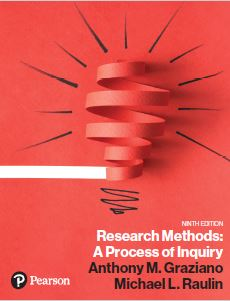﻿ Chapter 5 ResourcesGraziano & Raulin
Research Methods (9th edition)

## Resources for Chapter 5

### 05:01 SPSS for Windows Tutorial

This unit is a brief tutorial on using SPSS for Windows.

### 05:02SPSS for Window Inferential Statistical Analyses

This unit is the summary of inferential statistical analyses using SPSS for Windows.

### 05:03SPSS for Window Descriptive Statistics

This unit is the index of descriptive statistical procedures using SPSS for Windows.

### 05:04 Degrees of Freedom

This unit describes what is meant by degrees of freedom.

### 05:05 Why the Variance Works

This unit describes why the squared quantity of variance is so useful in statistical analyses.

### 05:06 Pearson Product-Moment Correlation Procedures

This unit shows how to compute a Pearson product-moment correlation using SPSS for Windows.

### 05:07 Spearman Rank-Order Correlation Procedures

This unit shows how to compute a Spearman rank-order correlation using SPSS for Windows.

### 05:08 Linear Regression

This unit provides a primer on linear regression, showing how SPSS for Windows can be used to compute a basic linear regression analysis.

### 05:09 Reliability Indices

This unit shows how to compute reliability indices using SPSS for Windows.

### 05:10 The Standard Normal Distribution

This unit shows how to convert a Z-score to a percentile rank using the Standard Normal Distribution.

### 05:11 Independent Samples t-test Computational Procedures

This unit shows how to compute a t-test for independent samples using SPSS for Windows.

### 05:12 Correlated t-test Computational Procedures

This unit shows how to compute a correlated t-test using SPSS for Windows.

### 05:13 ANOVA Computational Procedures

This unit shows how to compute a basic one-way ANOVA procedures using SPSS for Windows.

### 05:14 Effect Size

This unit describes how to compute effect size for a study.

### 05:15 Statistical Theory

This unit provides extensive coverage of statistical concepts, the logic behind them, and how they are used in research.